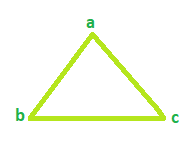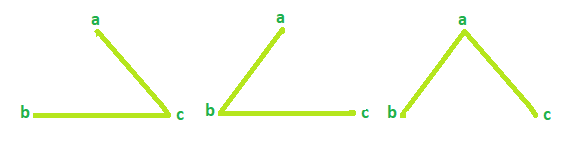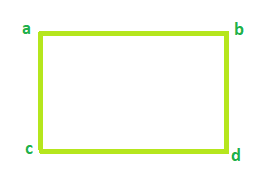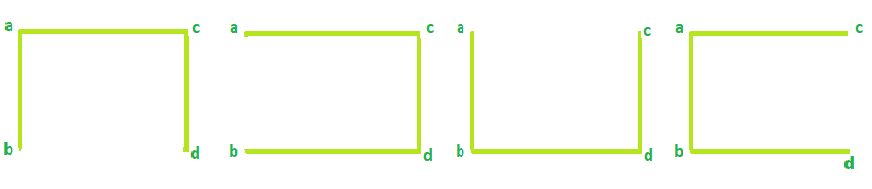Total number of Spanning trees in a Cycle Graph

• Difficulty Level : Basic
• Last Updated : 14 Apr, 2021

Given the number of vertices in a Cycle graph. The task is to find the Total number of Spanning trees possible.
Note: A cycle/circular graph is a graph that contains only one cycle. A spanning tree is a shortest/minimum path in a graph that covers all the vertices of a graph.

Examples:

Input: Vertices = 3
Output: Total Spanning tree = 3

Input: Vertices = 4
Output: Total Spanning tree = 4

Example 1:
For Cycle Graph with vertices = 3Spanning Tree possible is 3Example 2:
For Cycle Graph with vertices = 4Spanning Tree possible is 4So, the number of spanning treess will always be equal to the number of vertices in a cycle graph.

Below is the required implementation:

C++

 // C++ program to find number of // spanning trees #include using namespace std;   // function that calculates the // total Spanning tree int Spanning(int vertices) {     result = 0;       result = vertices;     return result; }   // Driver code int main() {     int vertices = 4;       cout << "Spanning tree = " << Spanning(vertices);     return 0; }

Java

 // Java program to find number of // spanning trees   import java.io.*;   class GFG {   // function that calculates the // total Spanning tree static int Spanning(int vertices) {     int result = 0;       result = vertices;     return result; }   // Driver code     public static void main (String[] args) {     int vertices = 4;       System.out.println("Spanning tree = " + Spanning(vertices));     } } // This code is contributed  // by chandan_jnu..

Python3

 # Python program to find number of # spanning trees   # function that calculates the # total Spanning tree def Spanning( vertices):         result = 0       result = vertices     return result   # Driver code vertices = 4 print("Spanning tree = ",        Spanning(vertices))   # This code is contributed # by Sanjit_Prasad

C#

 // C# program to find number // of spanning trees using System;   // function that calculates // the total Spanning tree class GFG { public int Spanning(int vertices) {     int result = 0;       result = vertices;     return result; }   // Driver code public static void Main() {     GFG g = new GFG();     int vertices = 4;       Console.WriteLine("Spanning tree = {0}",                         g.Spanning(vertices)); } }   // This code is contributed // by Soumik



Javascript



Output:

Spanning tree = 4

My Personal Notes arrow_drop_up
Recommended Articles
Page :